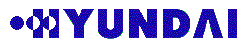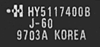Chipmunk International | Home | Technical Issues

If the information below appears garbled make sure that in the General Prreferences of Netscape the Fixed Font is set to Monaco or Courier.

## Understanding RAM chip numbers: HyundaiSample Explanation16 Mb Chip organised as: 4M x 4 bits
2K refresh, Fast Page Mode (FPM)
Speed = 60ns, Supply = 5 Volt

## Hyundai (HY) - 4 Megabit

How do I read the type number?

```HY514400-60
^^----------------- HY = Hyundai
HY514400-60
^^^-------------- 514 = 4  Megabit chip
511 = 16 Megabit chip: see below
531 = 1  Megabit chip
534 = 1  Megabit chip
53C = 256 Kilobit chip
HY514400-60
^^^----------- Internal Organization & Refresh:
100 = 4Meg x 1  / ??  refresh
260 = 256K x 16 / 512 refresh
400 = 1Meg x 4  / 1K  refresh
800 = 512K x 8  / 1K  refresh
HY514400-60
^^-------- Snelheid: 50=50ns / 60=60ns / 70=70ns / etc```

## Hyundai (HY) - 16 Megabit

How do I read the type number?

```HY51V17400BJ-60
^^----------------- HY = Hyundai
HY51V17400BJ-60
^^-^------------- 511 = 16 Megabit chip
514 = 4  Megabit chip: see above
516 = 64 Megabit chip
531 = 1  Megabit chip: see elsewhere
534 = 1  Megabit chip: see elsewhere
53C = 256 Kilobit chip: see elsewhere
HY51V17400BJ-60
^-------------- V = 3,3volt / (blank) = 5volt
HY51V17400BJ-60
^------------ 6 = 4K refresh
7 = 2K refresh
8 = 1K refresh
HY51V17400BJ-60
^^---------- Internal Organization:
10 = 16Meg x 1: check module size/banks
16 = 1Meg x 16: check module size/banks
40 = 4Meg x 4 : check module size/banks
HY51V17400BJ-60
^--------- 0 = Fast Page
4 = EDO
HY51V17400BJ-60
^^---- Speed: 50=50ns / 60=60ns / 70=70ns / etc```

## Hyundai (HY) - 64 Megabit

How do I read the type number?

```HY51V645400BJ-60
^^----------------- HY = Hyundai
HY51V645400BJ-60
^^--------------- 51 = DRAM chip
57 = SDRAM chip see below
HY51V645400BJ-60
^-------------- V = 3,3volt / (blank) = 5volt
HY51V645400BJ-60
^^------------ 64 = 64 Megabit chip (8K refresh)
65 = 64 Megabit chip (4K refresh)
1  = 16 Megabit chip: see above
4  = 4  Megabit chip: see above
HY51V645400BJ-60
^----------- 5 = ??  K refresh
HY51V645400BJ-60
^^--------- Internal Organization:
16 =
40 =
80 = 8Meg  x 8: check module size/banks
HY51V645400BJ-60
^-------- 0 = Fast Page
4 = EDO
HY51V645400BJ-60
^^--- Speed: 50=50ns / 60=60ns / 70=70ns / etc```

## Hyundai (HY) - SDRAM 64 Megabit

How do I read the type number?

```HY57V651620TC-10
^^----------------- HY = Hyundai
HY57V651620TC-10
^^--------------- 57 = SDRAM chip
HY57V651620TC-10
^-------------- V = 3,3volt / (blank) = 5volt
HY57V651620TC-10
^^------------ 16 = 16 Megabit chip
65 = 64 Megabit chip
HY57V651620TC-10
^^^^-------- Internal Organization:
1610 = 16 * 4K (2 bank)
1620 = 16Meg x 4 (4 bank)(64Mb chip) : check module size
4010 = 4 * 16K (2 bank)
4020 = 4 * 16K (4 bank)
8010 =  8 * 8K (2 bank)
8020 =  8 * 8K (4 bank) : check module size
HY57V651620TC-10
^^------ Speed:
TC  = PC66-222 specifications.  (only for old G3 series)
ATC  = PC100-323 specifications. (OK for Blue G3)
BTC   = PC100-222 specifications. (OK for Blue G3)
CTC   = PC100-222 specifications. (OK for Blue G3)
DTC   = PC100-222 specifications. (OK for Blue G3)
HY57V651620TC-10
^^--- Speed: 10```